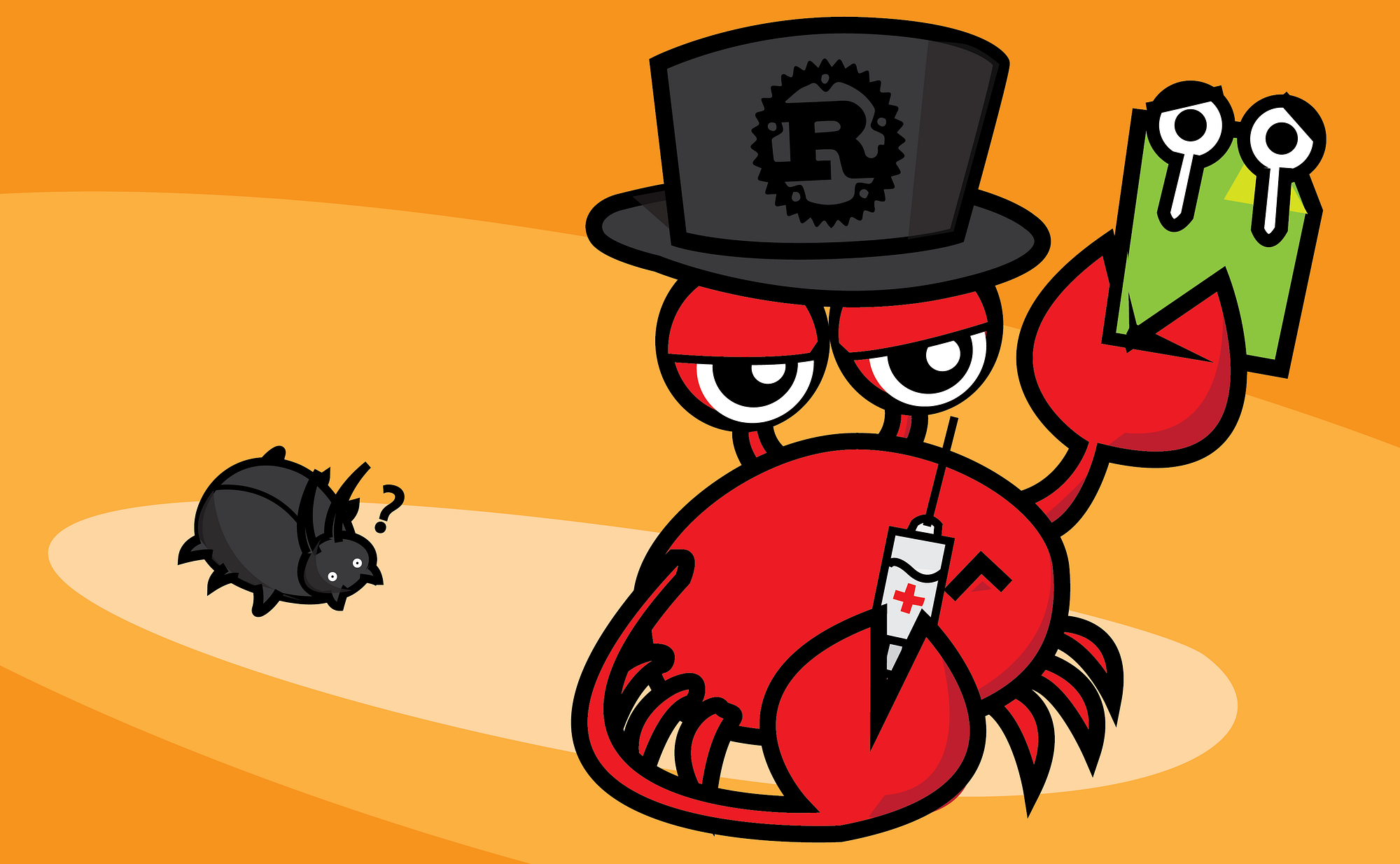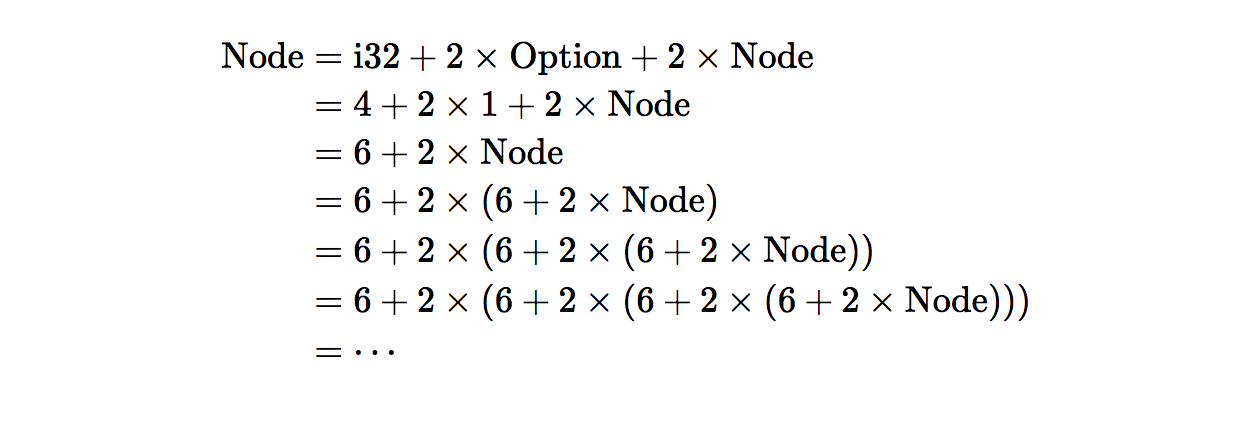# Recursive type problem in Rust

After spending much time reading about Rust, I decided to give it a try.It was a very simple implementation of Binary Tree Traversal algorithm. Surprisingly, by doing it, I learned a lot of Rust’s basic concepts!

## Rust tell us what’s wrong in our code

One of the first things that the smartass Rust compiler threw to my face was the lovely error message:

$rustc binary_tree.rs -o binary_tree error[E0072]: recursive type Node has infinite size --> binary_tree.rs:1:1 | | = help: insert indirection (e.g., a Box, Rc, or &) at some point to make Node representable error: aborting due to previous error  So yes, this is the code: struct Node { value: i32, left: Option<Node>, right: Option<Node> }  This code is a very obvious way to implement a binary tree node in other programming languages like C/C++ or Java. However, Rust compiler just doesn’t agree with us. Also, this is the interesting part. Take a closer look. The error message said that our Node struct is a recursive type and it has infinite size. What does it mean? In Rust, all values are allocated in stack by default. So the compiler needs to know the size of each. The size of a struct is the sum of all its fields size. For example, with this struct: struct Point { x: i32, y: u8 }  So the size of Point struct is: size_of::<Point>() == size_of::<i32>() + size_of::<u8>()  Back to our implementation, how do we calculate our Node’s size? size_of::<i32>() + 2 * size_of::<Option>() + 2 * size_of::<Node>()  Let’s expand this equation:Hey hey! Hey! Stop it! You can do this for all day long. There’s no way to stop the expanding process. So, the size of Node would be infinite and become impossible for Rust compiler to calculate. ## And Rust also tell us how to fix it Let’s take a look at the error message again. You can see the kindness of Rust when it tries to teach us how to repair the broken: $ rustc binary_tree.rs -o binary_tree

error[E0072]: recursive type Node has infinite size
--> binary_tree.rs:1:1
|
1 | struct Node {
| ^ recursive type has infinite size
|

error: aborting due to previous error


If we follow the hint and add Box to our implementation, the problem will be solved:

struct Node {
value: i32,
left: Option<Box<Node>>,
right: Option<Box<Node>>
}


However, what is Box? How does it solve our recursive reference problem?

Box is a pointer that pointed to a heap allocated memory space.

So when we declare a reference to Node using Box, the size of this reference is the size of a pointer, not the size of the Node type, and it is defined, so Rust compiler now aware how much memory needed to allocate for a Node. And the recursive type problem now solved!

I hope you enjoy the post and now understand the problem of recursive type, how to fix it.

Please feel free to leave a comment if you want to discuss or subscribe to my blog to keep updated about my next posts in Rust (and other technical stuff, of course).